# GMAT Math : Calculating the perimeter of a quadrilateral

## Example Questions

### Example Question #1 : Calculating The Perimeter Of A Quadrilateral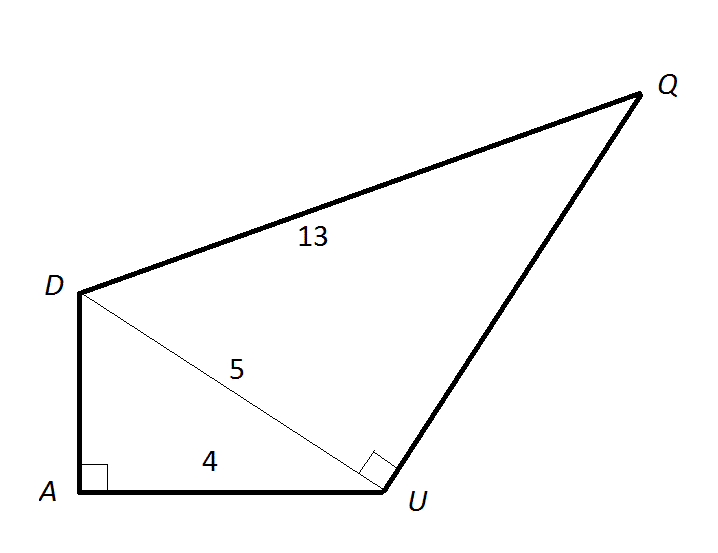Note: Figure NOT drawn to scale

What is the perimeter of Quadrilateral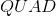, above?Explanation:

By the Pythagorean Theorem,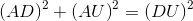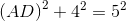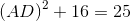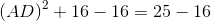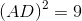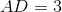Also by the Pythagorean Theorem,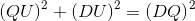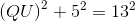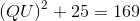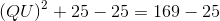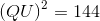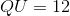The perimeter of Quadrilateralis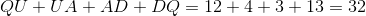### Example Question #2 : Calculating The Perimeter Of A Quadrilateral

What is the perimeter of Rhombus?

Statement 1: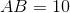Statement 2: Rhombushas area.

Statement 1 ALONE is sufficient to answer the question, but Statement 2 ALONE is NOT sufficient to answer the question.

BOTH statements TOGETHER are insufficient to answer the question.

Statement 2 ALONE is sufficient to answer the question, but Statement 1 ALONE is NOT sufficient to answer the question.

EITHER statement ALONE is sufficient to answer the question.

BOTH statements TOGETHER are sufficient to answer the question, but NEITHER statement ALONE is sufficient to answer the question.

Statement 1 ALONE is sufficient to answer the question, but Statement 2 ALONE is NOT sufficient to answer the question.

Explanation:

A rhombus has four congruent sides. Statement 1 gives the length of one of them, so that length can be multiplied byto yield the perimeter.

The area of a rhombus alone has no bearing on its perimeter, so Statement 2 alone is insufficient.

### Example Question #3 : Calculating The Perimeter Of A Quadrilateral

Isosceles trapezoid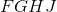has a side length of. The length of one of its bases (Base A) is equal to three times the amount of seven inches fewer than the length of the other base (Base B). If Base B has a length of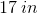, what is the perimeter of?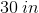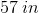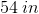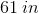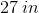Explanation:

In an isosceles trapezoid, the two non-parallel sides are equal. In this case, they are both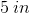long. To find the perimeter, we also need to know the length of both bases. We are told that Base B is. Eliminate any options less than or equal to.

To find the length of Base A, we need to translate the following: "The length of one of its bases (Base A) is equal to three times the amount of seven inches fewer than the length of the other base (Base B)." For translating, break the statement down:

Starting with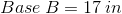,

"seven inches fewer than the length of the other base (Base B)":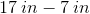"three times the amount of seven inches fewer than the length of the other base (Base B)":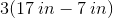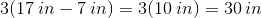So, Base A islong, so the perimeter of isosceles trapezoidis: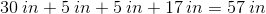### Example Question #4 : Calculating The Perimeter Of A Quadrilateral

Frank is planning on fencing a rectangular field near his house. The longer side of the house is two times four more than the length of the other side. If the shorter side ismeters, what is the total length of fence that Frank needs?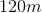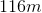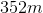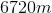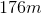Explanation:

This is a perimeter problem, but first we need to find our side lengths.

The short sides aremeters. The long sides are two times 4 more than the short sides. So

"four more"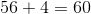"two times"meters

So our two side lengths are 120 and 56 meters. Find the perimeter by the following: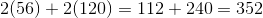So, 352 meters

### Example Question #5 : Calculating The Perimeter Of A Quadrilateral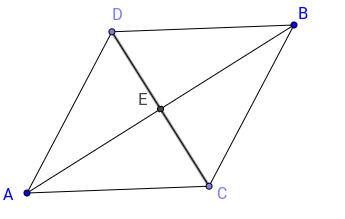Rhombus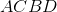has diagonals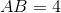and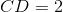. What is the perimeter of the rhombus?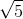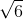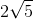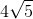The rhombus is a special kind of a parallelogram. Its sides are all of the same length. Therefore, we just need to find one length of this quadrilateral. To do so, we can apply the Pythagorean Theorem on triangle AEC for example, since we know the length of the diagonals. Also, the diagonals intersect at their center. Therefore, triangle AEC has length,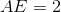and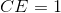. Therefore,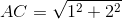or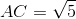. The perimeter is then.